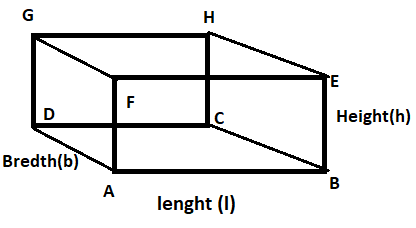Courses
Courses for Kids
Free study material
Offline Centres
MoreLast updated date: 24th Nov 2023
Total views: 379.8k
Views today: 5.79k

# If length, breadth and height of a cuboid are 20cm, 15cm and 10cm respectively, then find :(a) Area of the base.(b) Surface area of its vertical faces.(c) Volume.Verified
379.8k+ views
Hint:In order to solve this problem, we must use formulas of surface area and volume of Cuboid along with proper understanding of length, breadth and height of cuboid to get the required result.In the question it is given the length(l) = 20cm, breadth(b) =15cm, height(h)=10cm of cuboid.
(A) Area of base ABCD = length(l) × breadth(b)
So on putting the values of length(l) & breadth(b)
=20×15
=300${\text{c}}{{\text{m}}^2}$.

(B) Surface area of its vertical faces
Here it is total 4 vertical surfaces, ABEF, BEHC, HCDG, AFGD
By observing the given figure, we can say that Surface area of its vertical faces ABEF, HCDG will be equal and will be the length(l) × height(h)
So Surface area of its vertical faces ABEF, HCDG = 2 (length(l) × height(h))

Similarly, by observing the given figure, we can say that Surface area of its vertical faces BEHC, AFGD will be equal and will be the breadth(b) × height(h)
So Surface area of its vertical faces BEHC, AFGD = 2 (breadth(b) × height(h))

So Total Surface area of its vertical faces =
2 (length(l) × height(h)) + 2 (breadth(b) × height(h))
=2(lh+bh)
So on putting all the values
= 2(20×10+15×10)
=2×350
=700 ${\text{c}}{{\text{m}}^2}$.

(C) we know that the Volume of cuboid is equal to length(l) × breadth(b) × height(h)
So on putting all the value
=20×15×10
=300×10
=3000 ${\text{c}}{{\text{m}}^3}$.

Note: Whenever we face such types of problems the key concept we have to remember is that always remember the formula of Surface area and volume of Cuboid which are stated above, then using those formulas calculate, whatever is asked in question.